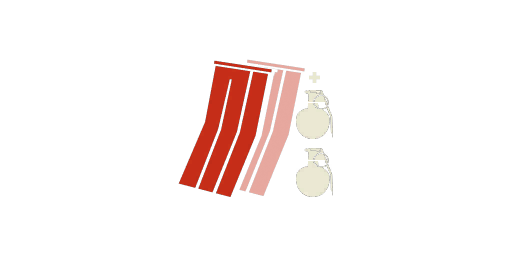The Chest Rig is a type of vest.

## Insurgency

{{#infoboxbuilder: | 0:Theme = INS default | 0:CustomModule = | 0:MainImageCaption = | 0:ToggleContentLongerThan = 1500

| 1:Type = Title

```| 1:Value = Chest Rig
```

| 2:Type = MainImage

```| 2:Value =```

| 3:Value =

General information

| 4:Type = Line

```| 4:Label = Inventory slot
| 4:Value = Vest
```

| 5:Type = Line

```| 5:Label = Weapon class
| 5:Value =
```

| 6:Type = Line

```| 6:Label = Fire modes
| 6:Value =
```

| 7:Type = Line

```| 7:Label = Operators
| 7:Value =
```

| 9:Type = Line

```| 9:Label = Console ?
| 9:Value =
```

| 10:Value =

Statistics
Damage

| 11:Type = Line

```| 11:Label = Damage
| 11:Value =
```

| 12:Type = Line

```| 12:Label = Explosion radius
| 12:Value =
```

| 14:Type = Line

```| 14:Label = Max range ?
| 14:Value =
```

| 15:Type = Line

```| 15:Label = Rate of fire
| 15:Value =
```

| 16:Type = Line

```| 16:Label = Fuse time
| 16:Value =
```

| 17:Type = Line

```| 17:Label = Cookable?
| 17:Value =
```

| 18:Type = Line

```| 18:Label = Effect duration
| 18:Value =
```

| 19:Value =

Ammunition

| 20:Type = Line

```| 20:Label = Cartridge
| 16:Value =
```

| 21:Type = Line

```| 21:Label = Magazine size
| 21:Value =
```

| 22:Type = Line

```| 22:Label = Starting ammo
| 22:Value =
```

| 23:Type = Line

```| 23:Label = Reload time
| 23:Value =
```

| 24:Value =

Handling

| 25:Type = Line

```| 25:Label = Recoil
| 25:Value =
```

| 26:Type = Line

```| 26:Label = Center speed
| 26:Value =
```

| 27:Type = Line

```| 27:Label = Spread
| 27:Value =
```

| 28:Type = Line

```| 28:Label = Idle sway
| 28:Value =
```

| 29:Value =

General statistics

| 30:Type = Line

```| 30:Label = Suppression
| 30:Value =
```

| 31:Type = Line

```| 31:Label = Penetration
| 31:Value =
```

| 32:Type = Line

```| 32:Label = Optic magnification
| 32:Value =
```

| 33:Type = Line

```| 33:Label = Supply points ?
| 33:Value = 1
```

| 34:Type = Line

```| 34:Label = Weight points ?
| 34:Value = 64/640
```

| 35:Type = Line

```| 35:Label = Weight
| 35:Value = 2.2kg
```

| 36:Type = Line

```| 36:Label = Length ?
| 36:Value =
```

}} The Chest Rig is an ammo vest featured in Insurgency. It is available to all factions and units.

It provides the user two extra magazines of primary ammo and secondary ammo. Additionally, it makes the secondary explosive slot available. Some explosives can only be equipped in the first explosives slot; therefore, [[::Category:Rockets|rocket launchers]], C4, and IEDs may not be granted more than one ammunition.